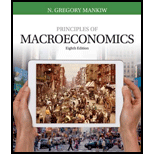# It is sometimes suggested that the Federal Reserve should try to achieve zero inflation. If we assume that velocity is constant, does this zero-inflation goal require that the rate of money growth equal zero? If yes, explain why. If no, explain what the rate of money growth should equal.### Principles of Macroeconomics (Mind...

8th Edition
N. Gregory Mankiw
Publisher: Cengage Learning
ISBN: 9781305971509### Principles of Macroeconomics (Mind...

8th Edition
N. Gregory Mankiw
Publisher: Cengage Learning
ISBN: 9781305971509

#### Solutions

Chapter
Section
Chapter 17, Problem 3PA
Textbook Problem
442 views

## It is sometimes suggested that the Federal Reserve should try to achieve zero inflation. If we assume that velocity is constant, does this zero-inflation goal require that the rate of money growth equal zero? If yes, explain why. If no, explain what the rate of money growth should equal.

Expert Solution
To determine
Zero inflation and rate of money growth.

### Explanation of Solution

By the quantity theory of money, achieving zero inflation rate would require the growth rate of quantity of money to be equal to the growth rate of output, as

### Want to see the full answer?

Check out a sample textbook solution.See solution

### Want to see this answer and more?

Bartleby provides explanations to thousands of textbook problems written by our experts, many with advanced degrees!

See solution

Find more solutions based on key concepts
Show solutions
What adverse effect might be caused by tax incentives to increase saving?

Brief Principles of Macroeconomics (MindTap Course List)